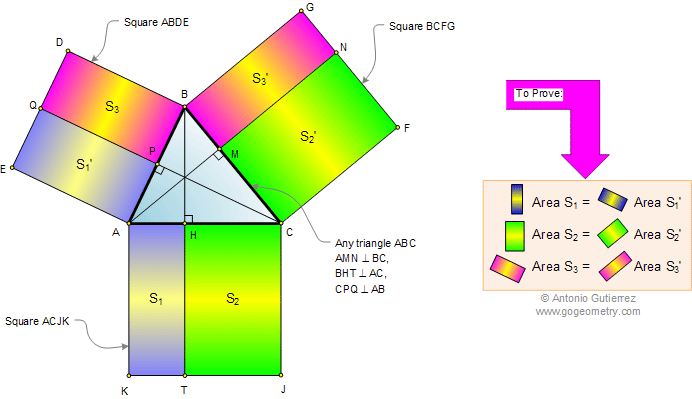Problem 521: Triangle, Squares, Altitude, Rectangles, Areas.
Level: High School, College, SAT Prep.

The drawing shows a triangle ABC with the squares ABDE, BCFG, and ACJK. Lines AMN, BHT, and CPQ are perpendicular to BC, AC, and AB respectively. Prove that the areas of the rectangles S1 and S1' are equal, S2 and S2' are equal, and S3 and S3' are equal.Geometry problem solving is one of the most challenging skills for students to learn. When a problem requires auxiliary construction, the difficulty of the problem increases drastically, perhaps because deciding which construction to make is an ill-structured problem. By “construction,” we mean adding geometric figures (points, lines, planes) to a problem figure that wasn’t mentioned as "given."